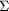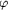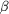# search results

## results of search on CRYSTALLOGRAPHY JOURNALS ONLINE

57 citations found for Langs, D.A.

There are 60 articles by Langs, D. click here to see these.

Search for Langs, D.A. in the World Directory of Crystallographers

Results 1 to 20, sorted by name:

### Statistical Expectation Value of the Debye-Waller Factor and E(hkl) Values for Macromolecular Crystals

The statistical evaluation of the Debye-Waller factor for a unit-cell distribution of atomic B values is used to improve intensity modfication for protein crystals.

### A priori estimation of scale and overall anisotropic temperature factors from the Patterson origin peak

An idea due to D. Rogers [Computing Methods in Crystallography (1965), edited by J. S. Rollett, pp. 117-148. Oxford: Pergamon Press] has been developed and implemented. The method is an advantageous alternative to Wilson plot or K-curve scaling of intensity data. On the relative experimental scale the structure factor can be written in matrix notation as F(h) = k-1jfj(h) exp (2ihTxj) exp (-hTbjh); and the squared structure-factor magnitude can be written as |F(h)|2 = k-2 exp (-2hTbh){jf2j + 2jk>jfjfk exp [2ihT(xj - xk)]}, if a common, or average, anisotropic temperature factor is factored out of the atomic summations. The fj2 summation corresponds to the Patterson origin peak, and the fj fk double summation to the off-origin Patterson peaks. A trivariate Gaussian density function, P(u) - Pmin = p0 exp (-uTpu), is fitted by least squares to the origin peak from a Patterson synthesis with coefficients |F|2meas/jf2j. Fourier inversion of the fitted Gaussian gives the scale and thermal parameters, k2 = (det p)1/2/(3/2VcellP0) and b = (2/2)p-1. The fit of the parameter Pmin is constrained by the condition that Pmin = -F(000)2/(k2 VcelljZ2j), and thus only P0 and the six coefficients Pij (i < j = 1, 2, 3) are independent parameters.

### Data averaging with normal down-weighting of outliers

A simple scheme is described for calculating weighted averages of multiple equivalent measurements using weights based on estimated normal probabilities.

### Use of negative quartet cosine invariants as a phasing figure of merit: NQEST

Recent theoretical advances in the identification of those cosine invariants cos (h +k +1 +m) which are probably negative suggest algorithms for the calculation of a figure of merit which is sensitive to the integrity of a phase set. The negative quartet figure of merit, NQEST, defined here is of particular utility in conjunction with fast multi-solution tangent formula techniques. Development of the methods and applications to both known and unknown crystal structures are presented.

### Prostadienoic acids PGE2 and PGF2: crystallographic studies of conformational transmission and receptor recognition

Acta Cryst. (2004). A60, s165

### Structure of gramicidin D-RbCl complex at atomic resolution from low-temperature synchrotron data: interactions of double-stranded gramicidin channel contents and cations with channel wall

A 1.14 Å resolution study on the gramicidin D complex with RbCl confirms its right-handed antiparallel double-stranded dimeric structure. Rb cations distributed over seven binding sites in each gramicidin channel unit are `coordinated' by delocalized-electrons of three to five carbonyl groups from the channel wall and by two water molecules.

### Globbic approximation in low-resolution direct-methods phasing

Probabilistic direct-methods phasing theory is shown to be adaptable to a non-uniform bulk-solvent-compensated approximation for protein crystals at low resolution.

### Use of globic scattering factors for protein structures at low resolution

At 3 to 4 Å resolution, the electron density of a protein may be modeled by a continuous chain of `globs' representing the amide region of the peptide backbone and the side-chain residues. Group scattering factors are derived from a trans planar CC=ONCbackbone segment and most favored side-chain conformer for 18 different amino acids. Trial calculations indicate that the phase error and crystallographic residual comparing the atomic and `globic' models rapidly decrease from high to low resolution. At 3 Å resolution, the phase error is approximately 80°. These results indicate that the electron density of a protein composed of N amino acid residues may be adequately modeled by 2N globs at low resolution.

### On `globbicity' of low-resolution protein structures

Globbic scattering factors have been calculated for main-chain peptide units and amino-acid side-chain groups to 3 Å resolution via Debye's formula. They are also approximated by a single-Gaussian formula.

Acta Cryst. (1996). A52, C73

### On Integrating the Techniques of Direct Methods with Anomalous Dispersion. IV. A Simplified Perturbation Treatment for SAS Phasing

A treatment based on Friedel-pair two-phase invariants shows that single-wavelength anomalous-scattering (SAS) three-phase invariants tend to positive values and yields an origin-shifted tangent formula.

### Bulk-solvent correction in direct-methods phasing

An algebraic analysis leads to bulk-solvent-corrected forms of the Sayre equation and tangent formula, which are verified by empirical numerical calculations for two protein structures with molecular weights of about 100 and 150 kDa.

### Direct methods: the identification of space-group-specific inconsistent three-phase structure invariants

Certain space groups often permit the generation of pairs of triple relationships involving the same three parent reflections in different symmetry forms, giving rise to two equally probable invariant estimates which, because of the space-group symmetry, must disagree by an a priori known phase shift. The 230 space groups have been examined to identify those which permit inconsistent triples, and the complete list which describes the forms of the pair of triples and their phase inconsistency is given.

### The phase problem in neutron crystallography

The phase problem of neutron crystallography is formulated as a problem in constrained global minimization, the solution of which, by means of a modified Shake-and-Bake algorithm (Neutron Shake-and-Bake), exploits the property of the neutron density function that it takes negative as well as positive values. The applications show that the positivity of the density function is not only not required for the solution of the phase problem but that positivity is actually a hindrance.

### An efficient molecular-replacement translation function based on the evaluation of direct-methods phase invariants

Traditional molecular-replacement translation functions are based on direct- or reciprocal-space correlations between the observed diffraction amplitudes and the calculated amplitudes and phases of the symmetry-related molecular transforms of the search fragment as a function of the displacement vector. An alternative method that has been described is based on evaluating a list of phase invariants as a function of the position of the search model in the unit cell and seeking those regions which satisfy the expectation value of these invariants as predicted by probability theory. As originally formulated, this procedure required the iterative computation of the phases and the evaluation of the list of invariants as the search model was stepped over the grid points defining the asymmetric portion of the unit cell. A new computational procedure is described whereby the values of the invariants are expressed solely as a function of the displacement vector r as a Fourier series that can be evaluated by a standard fast Fourier transform (FFT) without having to compute and insert the values of the phases based on the search model at each grid point.

Acta Cryst. (1984). A40, C83